# Math Olympiad Test: Quadratic Equations- 1

Test Description

## 10 Questions MCQ Test International Mathematics Olympiad (IMO) for Class 10 | Math Olympiad Test: Quadratic Equations- 1

Math Olympiad Test: Quadratic Equations- 1 for Class 10 2022 is part of International Mathematics Olympiad (IMO) for Class 10 preparation. The Math Olympiad Test: Quadratic Equations- 1 questions and answers have been prepared according to the Class 10 exam syllabus.The Math Olympiad Test: Quadratic Equations- 1 MCQs are made for Class 10 2022 Exam. Find important definitions, questions, notes, meanings, examples, exercises, MCQs and online tests for Math Olympiad Test: Quadratic Equations- 1 below.
Solutions of Math Olympiad Test: Quadratic Equations- 1 questions in English are available as part of our International Mathematics Olympiad (IMO) for Class 10 for Class 10 & Math Olympiad Test: Quadratic Equations- 1 solutions in Hindi for International Mathematics Olympiad (IMO) for Class 10 course. Download more important topics, notes, lectures and mock test series for Class 10 Exam by signing up for free. Attempt Math Olympiad Test: Quadratic Equations- 1 | 10 questions in 10 minutes | Mock test for Class 10 preparation | Free important questions MCQ to study International Mathematics Olympiad (IMO) for Class 10 for Class 10 Exam | Download free PDF with solutions
 1 Crore+ students have signed up on EduRev. Have you?
Math Olympiad Test: Quadratic Equations- 1 - Question 1

### The area of a square is 169 cm2. What is the length of one side of the square?

Detailed Solution for Math Olympiad Test: Quadratic Equations- 1 - Question 1

Since a square has sides with the same length, the formula for Area is A = s2
Let’s substitute 169 for the Area.
s2 = A
s2 = 169
s = √169
s = 13
In this situation, -13 doesn’t apply since the side of a square can’t be a negative number.

Math Olympiad Test: Quadratic Equations- 1 - Question 2

### A right triangle has a side with length 12 in and a hypotenuse with length 20 in. Find the length of the second leg. (Round to the nearest hundredth if needed)

Detailed Solution for Math Olympiad Test: Quadratic Equations- 1 - Question 2

Since we are working with a right triangle, and we are missing a leg, we can use the Pythagorean Theorem to solve for that missing leg
Pythagorean Theorem: A2 + B2 = C2
A = 12, B = ?, C = 20
122 + B2 = 202 Substitute
144 + B2 = 400 Simplify
144 - 144 + B2 = 400 - 144
Subtract 144 from both sides
B2 = 256 Simplify
Take the square root of both sides
B = 16

Math Olympiad Test: Quadratic Equations- 1 - Question 3

### Find the x-intercepts for the following equation. Y =  6x2 - 7x - 3

Detailed Solution for Math Olympiad Test: Quadratic Equations- 1 - Question 3

In order to find the x-intercepts, we can let y = 0 and solve for x. We can solve by possible factoring or by using the quadratic formula. Let’s use the discriminant to decide which method would be best.
0 = 6x2 - 7x - 3
where a = 6
b = -7
c = -3
Discriminate: b2 - 4ac
(-7)2 - 4(6)(-3) = 121.
This is positive and a perfect square, so let’s factor!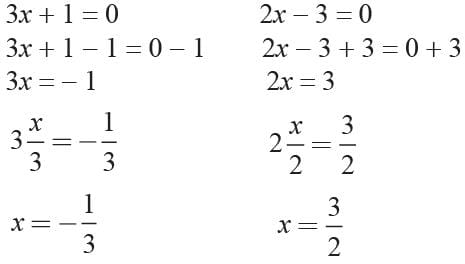Math Olympiad Test: Quadratic Equations- 1 - Question 4

Use the quadratic formula to find the values of x for the equation:
x2 - 4x - 10 = 0

Detailed Solution for Math Olympiad Test: Quadratic Equations- 1 - Question 4

Given a quadratic equation of the form
ax2 + bx + c = 0
the quadratic roots can be evaluated using the formula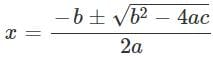For x2 − 4x + 10 = 0
∴ x = 5.74 and x = -1.74

Math Olympiad Test: Quadratic Equations- 1 - Question 5

Which statement best describes the solutions to the equation below?
3x2 - 5x + 20 = 0

Detailed Solution for Math Olympiad Test: Quadratic Equations- 1 - Question 5

In order to determine which statement best represents this equation, I would need to find the discriminate. Discriminate: b2 - 4ac
where: a = 3 b = -5 c = 20
(-5)2 - 4(3) (20) = -215
Since the discriminate is negative, this means that there are no real solutions.

Math Olympiad Test: Quadratic Equations- 1 - Question 6

Solution set of 6x2 + x - 15 = 0 is

Detailed Solution for Math Olympiad Test: Quadratic Equations- 1 - Question 6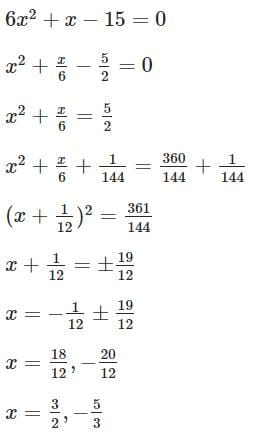Math Olympiad Test: Quadratic Equations- 1 - Question 7

Graph of quadratic function is

Detailed Solution for Math Olympiad Test: Quadratic Equations- 1 - Question 7

The graph of a quadratic function is called a parabola.

Math Olympiad Test: Quadratic Equations- 1 - Question 8

If -5, is a root of the quadratic equation 2x2 + Px - 15 = 0 and the quadratic equation P (x2 +x) + K = 0 has equal roots. What is the value of K?

Detailed Solution for Math Olympiad Test: Quadratic Equations- 1 - Question 8

The given equation is
2x2 + Px - 15 = 0
2(-5)2 + P(-5) - 15 = 0
⇒ 50 - 5P - 15 = 0
⇒ -5P = - 35
⇒ P = 7
P(x2 + x) + K = 0
⇒ 7(x2 + x) + K = 0 ⇒ 7x2 + 7x + K = 0
⇒ a = 7, b = 7, c = K
∴ D = b2 - 4ac = (7)2 - 4(7)K = 49 - 28K
The equation has equal roots
D = 0 ⇒ 49 - 28K = 0 ⇒ K = 49/28 = 7/4

Math Olympiad Test: Quadratic Equations- 1 - Question 9

If one root of 3x2 + 11x +K = 0 is reciprocal of the other then what is the value of K?

Detailed Solution for Math Olympiad Test: Quadratic Equations- 1 - Question 9

The given equation is
3x2 + 11x + K = 0
Let one root be α then other root is 1/α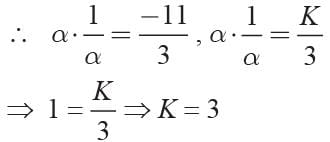Math Olympiad Test: Quadratic Equations- 1 - Question 10

If α, β are the roots of the equation 3x2 + 8x + 2 = 0 then the value of (1/α + 1/β) is

Detailed Solution for Math Olympiad Test: Quadratic Equations- 1 - Question 10

We have 3x2 + 8x + 2 = 0
and α + β = -8/3, αβ = 2/3
Now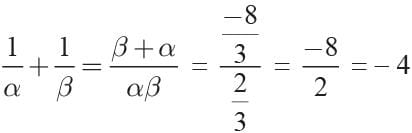## International Mathematics Olympiad (IMO) for Class 10

22 docs|62 tests
 Use Code STAYHOME200 and get INR 200 additional OFF Use Coupon Code